Updating search results...

# 61 Results

View
Selected filters:
• tmm0022Conditional Remix & Share Permitted
CC BY-NC
Rating
0.0 stars

TMM 002 PRECALCULUS (Revised March 21, 2017)3.&nbsp; Equations and Inequalities:&nbsp; &nbsp;&nbsp; 3f.&nbsp; Solve systems of equations using substitution and/or elimination.*

Subject:
Mathematics
Material Type:
Module
05/28/2019Conditional Remix & Share Permitted
CC BY-NC
Rating
0.0 stars

TMM 002 PRECALCULUS (Revised March 21, 2017)3.&nbsp; Equations and Inequalities:&nbsp; &nbsp;&nbsp; 3a.&nbsp; Recognize function families as they appear in equations and inequalities and choose an appropriate solution methodology for a particular equation or inequality, as well as communicate reasons for that choice.*

Subject:
Mathematics
Material Type:
Module
05/28/2019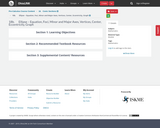Conditional Remix & Share Permitted
CC BY-NC
Rating
0.0 stars

Ellipse - conic section, foci, major and minor axes, vertices, standard form, eccentricityTMM 002 PRECALCULUS (Revised March 21, 2017)AdditionalOptional Learning Outcomes:2. Geometry: The successful Precalculus student can:2f. Represent conic sections algebraically via equations of two variables and graphically by drawing curves.Sample Tasks:The student can perform the process &ldquo;completing the square&rdquo; transforming the equation into a standard form.The student can draw curves representing conic sections.The student can solve systems of equations involving linear and quadratic functions.The student can parametrize conic curves.

Subject:
Algebra
Astronomy
Geometry
Higher Education
Mathematics
Material Type:
Module
05/28/2019Conditional Remix & Share Permitted
CC BY-NC
Rating
0.0 stars

Hyperbola - conic section, foci, transverse and conjugate axes, vertices, asymptotes, standard formTMM 002 PRECALCULUS (Revised March 21, 2017)AdditionalOptional Learning Outcomes:2. Geometry: The successful Precalculus student can:2f. Represent conic sections algebraically via equations of two variables and graphically by drawing curves.Sample Tasks:The student can perform the process &ldquo;completing the square&rdquo; transforming the equation into a standard form.The student can draw curves representing conic sections.The student can solve systems of equations involving linear and quadratic functions.The student can parametrize conic curves.

Subject:
Astronomy
Geometry
Higher Education
Mathematics
Material Type:
Module
05/28/2019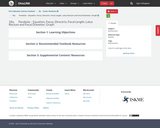Conditional Remix & Share Permitted
CC BY-NC
Rating
0.0 stars

Parabola - conic section, focus, directrix, focal length, standard form, vertex form, completing the square method.TMM 002 PRECALCULUS (Revised March 21, 2017)AdditionalOptional Learning Outcomes:2. Geometry: The successful Precalculus student can:2f. Represent conic sections algebraically via equations of two variables and graphically by drawing curves.Sample Tasks:The student can perform the process &ldquo;completing the square&rdquo; transforming the equation into a standard form.The student can draw curves representing conic sections.The student can solve systems of equations involving linear and quadratic functions.The student can parametrize conic curves.

Subject:
Algebra
Geometry
Higher Education
Mathematics
Material Type:
Module
05/28/2019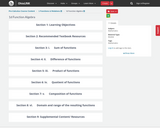Conditional Remix & Share Permitted
CC BY-NC
Rating
0.0 stars
Rating
0.0 stars
Subject:
Mathematics
Material Type:
Module
05/21/2019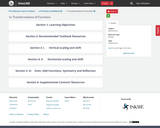Conditional Remix & Share Permitted
CC BY-NC
Rating
0.0 stars
Rating
0.0 stars
Subject:
Mathematics
Material Type:
Module
05/21/2019Conditional Remix & Share Permitted
CC BY-NC
Rating
0.0 stars
Rating
0.0 stars
Subject:
Mathematics
Material Type:
Module
05/21/2019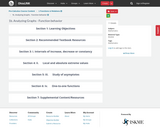Conditional Remix & Share Permitted
CC BY-NC
Rating
0.0 stars
Rating
0.0 stars
Subject:
Mathematics
Material Type:
Module
05/21/2019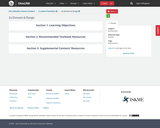Conditional Remix & Share Permitted
CC BY-NC
Rating
0.0 stars
Rating
0.0 stars
Subject:
Mathematics
Material Type:
Module
05/28/2019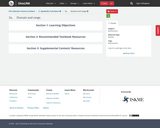Conditional Remix & Share Permitted
CC BY-NC
Rating
0.0 stars
Rating
0.0 stars
Subject:
Mathematics
Material Type:
Module
05/28/2019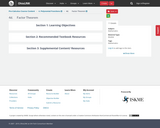Conditional Remix & Share Permitted
CC BY-NC
Rating
0.0 stars
Rating
0.0 stars
Subject:
Mathematics
Material Type:
Module
05/28/2019Conditional Remix & Share Permitted
CC BY-NC
Rating
0.0 stars
Rating
0.0 stars
Subject:
Mathematics
Material Type:
Module
05/28/2019Conditional Remix & Share Permitted
CC BY-NC
Rating
0.0 stars
Rating
0.0 stars
Subject:
Mathematics
Material Type:
Module
05/28/2019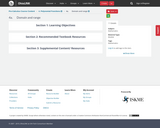Conditional Remix & Share Permitted
CC BY-NC
Rating
0.0 stars
Rating
0.0 stars
Subject:
Mathematics
Material Type:
Module